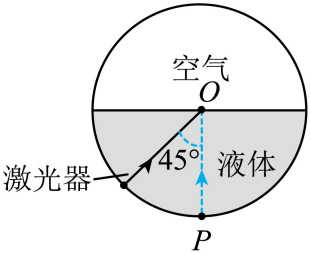$$\sin C=\frac{1}{n}$$

$$n=\frac{1}{\sin C}=\frac{1}{\sin 45^{\circ}}=\sqrt{2}$$

$$n=\frac{c}{v}$$

$$v=\frac{c}{n}=\frac{\sqrt{2}}{2} c$$